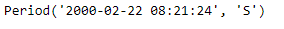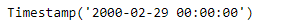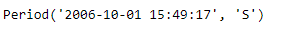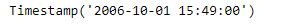# Python | Pandas Period.to_timestamp

• Last Updated : 06 Jan, 2019

Python is a great language for doing data analysis, primarily because of the fantastic ecosystem of data-centric python packages. Pandas is one of those packages and makes importing and analyzing data much easier.

Pandas` Period.to_timestamp()` function return the Timestamp representation of the Period at the target frequency at the specified end (how) of the Period.

Attention geek! Strengthen your foundations with the Python Programming Foundation Course and learn the basics.

To begin with, your interview preparations Enhance your Data Structures concepts with the Python DS Course. And to begin with your Machine Learning Journey, join the Machine Learning - Basic Level Course

Syntax : Period.to_timestamp()

Parameters :
freq : Target frequency. Default is ‘D’ if self.freq is week or longer and ‘S’ otherwise
how : ‘S’, ‘E’. Can be aliased as case insensitive ‘Start’, ‘Finish’, ‘Begin’, ‘End’

Return : Timestamp

Example #1: Use `Period.to_timestamp()` function to return the given period object as a Timestamp object in the specified frequency.

 `# importing pandas as pd``import` `pandas as pd`` ` `# Create the Period object``prd ``=` `pd.Period(freq ``=``'S'``, year ``=` `2000``, month ``=` `2``, day ``=` `22``,``                         ``hour ``=` `8``, minute ``=` `21``, second ``=` `24``)`` ` `# Print the Period object``print``(prd)`

Output :Now we will use the `Period.to_timestamp()` function to return the given period object as a timestamp object.

 `# return as a timestamp in the specified frequency.``# 'M' represents monthly frequency``prd.to_timestamp(freq ``=``'M'``)`

Output :As we can see in the output, the `Period.to_timestamp()` function has returned the given period object as a timestamp in the specified frequency.

Example #2: Use `Period.to_timestamp()` function to return the given period object as a Timestamp object in the specified frequency.

 `# importing pandas as pd``import` `pandas as pd`` ` `# Create the Period object``prd ``=` `pd.Period(freq ``=``'S'``, year ``=` `2006``, month ``=` `10``,``               ``hour ``=` `15``, minute ``=` `49``, second ``=` `17``)`` ` `# Print the object``print``(prd)`

Output :Now we will use the `Period.to_timestamp()` function to return the given period object as a timestamp object.

 `# return as a timestamp in the specified frequency.``# 'T' represents minutely frequency``prd.to_timestamp(freq ``=``'T'``)`

Output :As we can see in the output, the `Period.to_timestamp()` function has returned the given period object as a timestamp in the specified frequency.

My Personal Notes arrow_drop_up